Practice adding 4-digit numbers with these printable worksheets. Recommended for 3rd and 4th grade students.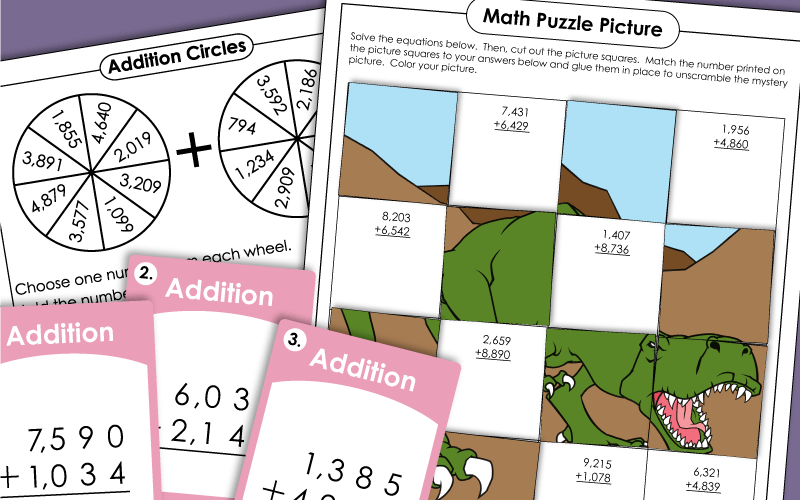Add the 4-digit addends to find the sums. Includes two word problems.
This worksheet includes a variety of 4-digit addition problems, including two word problems, horizontal and vertical equations, and more.
Students add 4-digit numbers to and 4-digit numbers. The graph paper ensures that their columns are lined up correctly.
Add four-digit numbers to complete this math crossword puzzle.
At the top of this page are numbers written inside of polygons. Students add numbers in similar shapes. For example: Find the sum of the numbers in the octagons.
These cards have addition problems with 4-digit addends. Use these cars in your math learning center, with your classroom document camera, or as cards for math games.
Use the key to decode the addends in the secret symbol numbers. Then solve the 4-digit addition problems.
Look carefully at the completed addition problems. There are mistakes. Re-solve each problem to find the correct sum. Then explain the mistakes in the original solutions.
Find the sums. Then cut out the numbered puzzle pieces. Match each puzzle piece (sum) with the correct addition problem.
First solve the 4-digit plus 4-digit problems. Then glue the picture puzzle pieces over the answers to make a picture of a rocket ship blasting through outer space.
Solve the 4-digit plus 4-digit addition problems. Glue the answer squares on the grid to make a dinosaur picture.
These four-digit addition problems are written horizontally. Students rewrite them vertically to solve.
Solve each 4-digit addition problem. Then cut out the cards and sort them into two different categories: Odd Sums and Even Sums.
Choose numbers from each circle. Add them together to find the sums. Do this six times.
Write each number in expanded form. (example: 4,506 = 4,000 + 500 + 6)

Here's a riddle for students to solve: What do you call a skeleton that won't work? In order to solve the riddle, your students will need to solve these 4-digit column addition problems.
This page has 10 practice problems and a word problem. All are written vertically. The top of the page has an airplane picture. At the bottom there's a travel-themed word problem.
Each of the problems on this page have 4-digit numbers. Some problems have 3 addends, but most have 4. This activity has a picture of a satellite and a space-themed word problem.
Study the completed math problems and look for errors. Explain the mistakes and solve correctly.

Why are there fences around graveyards? To discover the answer to this math riddle, students will answer a series of mental math addition problems. Addends are multiples of 10, 100, or 1,000. (example: 4,000+2,000)

What did the duck eat for lunch? To find the answer to the riddle, solve the math problems. Then match the answers with the letters.
What do you do if you meet a blue elephant? To find out the answer to this riddle, solve the 5-digit addition problems.
This page contains tape diagrams (also known as bar models). Students will need to add or subtract to find the missing numbers.
Complete seven tape diagrams (bar models). Then write an addition and subtraction number sentence for each. This version is a 2-page worksheet with work space on the right.
This sheet has six bar models. Students add or subtract to find the missing numbers. This worksheet has mostly 5-digit numbers.
This page has 5-digit addition-subtraction tape diagrams (aka bar models). There are seven problems on this file.

Custom Math Worksheet
Generator Tool

Try making your own addition worksheets. Select the number of digits you'd like, and the number of problems. Our generator tool will make a worksheet specially for you.

Practice adding money amounts on these math printables. (Estimated levels: 3rd, 4th, and 5th grades)

If 4-digits is a bit too much for your students, try jumping down to the 3-digit addition PDFs.

## Sample Worksheet Images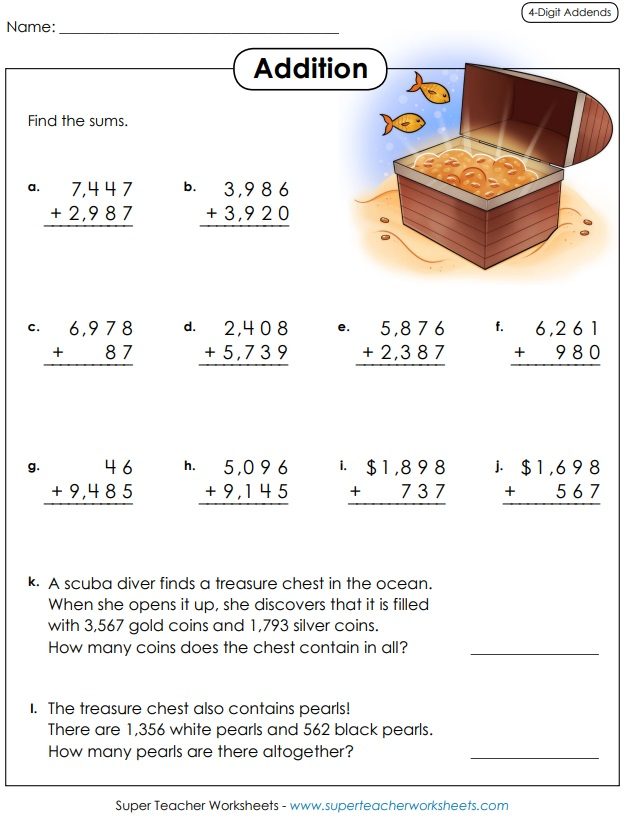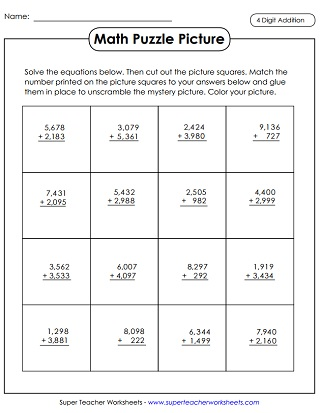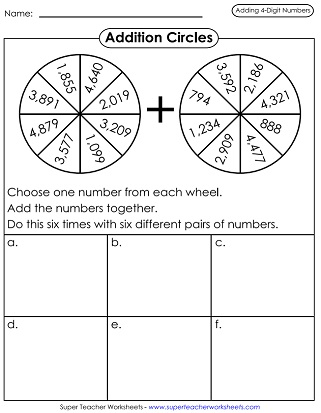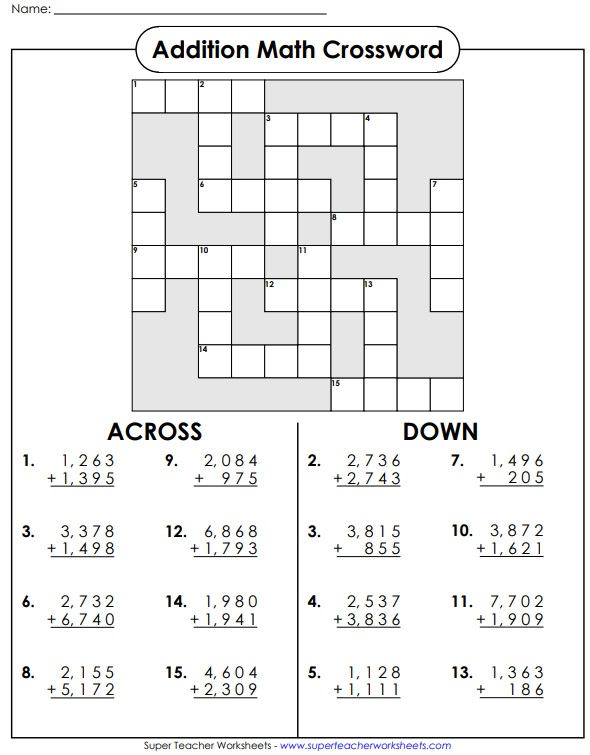My Account
Site Information